# Mathematics

### Chapter : Polynomials

#### To Form a Quadratic polynomial With Given Roots

Let zeros or roots of a quadratic quadrilateral be a and b

Example: Form the quadratic polynomial whose zeroes or roots are 4 and 6.

Solution: Sum of the zeroes = 4 + 6 = 10
Product of the zeroes = 4 × 6 = 24

Hence the polynomial formed by the given equation
= x2 – (sum of zeroes) x + Product of zeroes
= x2 – 10x + 24

Example: Form the quadratic polynomial whose zeroes are –3, 5.

Solution: Here, zeroes are – 3 and 5.
Sum of the zeroes = – 3 + 5 = 2
Product of the zeroes = (–3) × 5 = – 15

Hence the polynomial formed by
= x2 – (sum of zeroes) x + Product of zeroes
= x2 – 2x – 15

Example: Find a quadratic polynomial whose sum of zeroes and product of zeroes are respectively (i), –1 (ii) √2,(iii) 0, √5

Solution: Let the polynomial be ax2 + bx + c and its zeroes be a and b.

(i) Here, α + β =and α . β = – 1, therefore, the polynomial equation is formed by the formula
= x2 – (Sum of zeroes) x + Product of zeroes
= x2 –x – 1
The other polynomial are k.
If k = 4, then the polynomial is 4x2 – x – 4.

(ii) Here, α + β = √2 , α . β =. Thus the polynomial formed by
= x2 – (Sum of zeroes) x + Product of zeroes
= x2 – (√2) x +Other polynomial are k.
If k = 3, then the polynomial is 3x2 – 3√2x + 1

(iii) Here, α + β = 0 and α . β = √5, Thus the polynomial formed
= x2 – (Sum of zeroes) x + Product of zeroes
= x2 – (0) x + √5 = x2 + √5

Example: Find a cubic polynomial with the sum of its zeroes, sum of the products of its zeroes taken two at a time, and product of its zeroes as 2, – 7 and –14, respectively.

Solution: Let the cubic polynomial be ax3 + bx2 + cx + d, divide the whole polynomial by a, we get
⇒ x3 +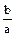x2 +x +....(1) and its zeroes are a, b and g, then
a + b + g = 2 = –ab + bg + ag = – 7 =abg = – 14 = –Putting the values of,and, in equtaion number 1, we get the cubic polynomial equation is
x3 + (–2) x2 + (–7)x + 14
⇒ x3 – 2x2 – 7x + 14

Example: Find the cubic polynomial with the sum, sum of the product of its zeroes taken two at a time and product of its zeroes as 0, –7 and –6 respectively.

Solution: Let the cubic polynomial be ax3 + bx2 + cx + d, then
⇒ x3 +x2 +x +....(1) and its zeroes are a, b, g. Then
a + b + g = 0 = –ab + bg + ag = – 7 =abg = – 6 = –Putting the values of,and,, and in (1), we get
x3 – (0) x2 + (–7) x + (–6)
or x3 – 7x + 6

Example: If a and b are the zeroes of the polynomials ax2 + bx + c then form the polynomial whose zeroes are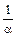and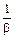.

Solution: Since a and b are the zeroes of ax2 + bx + c
So a + b =, ab =Sum of the zeroes =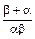=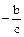Product of the zeroes
=×=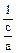But required polynomial is given by the equation x2 – (sum of zeroes) x + Product of zeroes
⇒ x2 –x +⇒ cExample: If a and b are the zeroes of the polynomial x2 + 4x + 3, form the polynomial whose zeroes are 1 +and 1 +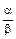.

Solution: Since a and b are the zeroes of the polynomial x2 + 4x + 3. Then, a + b = – 4, ab = 3

Sum of the zeroes
= 1 ++ 1 +=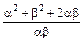=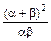==Product of the zeroes
= (1 +)(1 +)= 1 +++ 1
= 2 +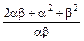===But required polynomial is given by the quadratic formula
x2 – (sum of zeroes) x + product of zeroes
or x2 –x +or kor 3(if k = 3)
⇒ 3x2 – 16x + 16